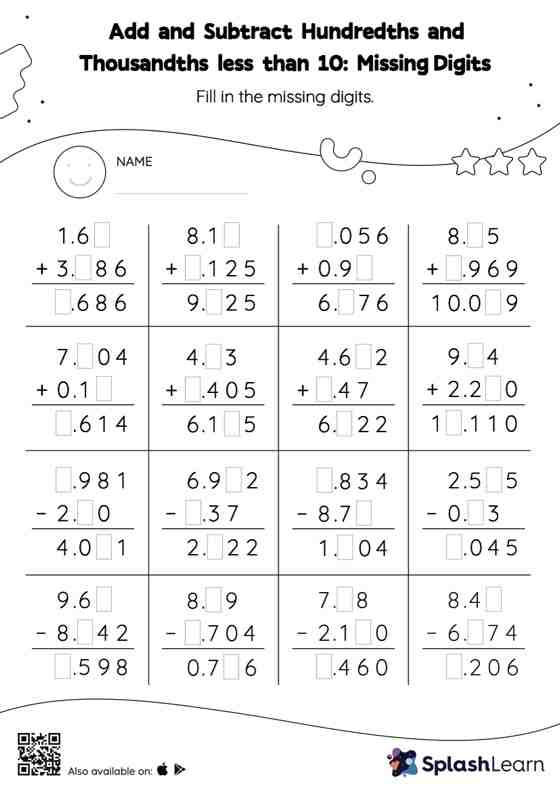# Add and Subtract Hundredths and Thousandths less than 10: Missing Digits Worksheet

Home > Add and Subtract Hundredths and Thousandths less than 10: Missing DigitsWhen adding or subtracting decimals on this worksheet, students align the decimal points and use zero as a placeholder. Then to find the missing number in the add and subtract hundredths and thousandths less than 10 worksheet, students then apply the relationship between addition and subtraction. This worksheet is about practicing the column method in which numbers are written one on top of another. In this method, students use their place value understanding to solve the problems.